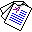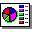# Brave Induction

## Chiaki Sakama and Katsumi Inoue

Proceedings of the 18th International Conference on Inductive Logic Programming (ILP'08), Lecture Notes in Artificial Intelligence 5194, pages 261-278, Springer-Verlag, 2008.

## Abstract

This paper considers the following induction problem. Given the background knowledge B and an observation O, find a hypothesis H such that a consistent theory B∧ H has a minimal model satisfying O. We call this type of induction brave induction. Brave induction is different from explanatory induction in ILP, which requires that O is satisfied in every model of B∧ H. Brave induction is useful for learning disjunctive rules from observations, or learning from the background knowledge containing indefinite or incomplete information. We develop an algorithm for computing brave induction, and extend it to induction in answer set programming.Full Paper (pdf 180K) ,Slide (pdf 271K) © Springer-Verlag